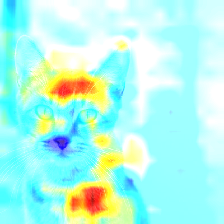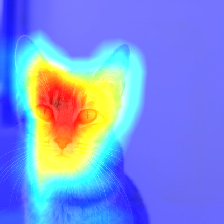## 11.5 Tool: tf-explain

tf-explain implements interpretability methods as Tensorflow 2.x callbacks to ease neural network’s understanding. See Introducing tf-explain, Interpretability for Tensorflow 2.0

The code can be found at GitHub

tf-explain:

• Implements interpretability methods as Tensorflow 2.x callbacks
• Several methods to look into different aspects
• Activations Visualization
• Occlusion Sensitivity
• Grad CAM (Class Activation Maps)

Visualize gradients on the inputs towards the decision.Occlusion Sensitivity

Visualize how parts of the image affects neural network’s confidence by occluding parts iterativelyVisualize how parts of the image affects neural network’s output by looking into the activation maps